# Variable Temperature and Concentration Impacts on Radiative Chemically Magnetohydrodynamic Viscoelastic Fluid Flow Through Porous Moving Plate

Variable Temperature and Concentration Impacts on Radiative Chemically Magnetohydrodynamic Viscoelastic Fluid Flow Through Porous Moving Plate

Karna Suneetha Shaik Mohammed IbrahimGurram Venkata Ramana Reddy Prathi Vijaya Kumar

Department of Mathematics, K. L. E. F, Vaddeswaram, Guntur, Andhra Pradesh 522502, India

Department of Applied Mathematics, GITAM Deemed to be University, Andhra Pradesh 530045, India

Corresponding Author Email:
sibrahim@gitam.edu
Page:
187-195
|
DOI:
https://doi.org/10.18280/mmep.070203
2 October 2019
|
Accepted:
11 November 2019
|
Published:
30 June 2020
| Citation

OPEN ACCESS

Abstract:

The flow has been made by considering variable temperature and radiation effects for the magnetohydrodynamic viscoelastic fluid past a moving vertical plate in a porous medium. Chemical reaction and concentration have been taken into account. The governed mathematical statement is handled analytically by perturbation technique. The main view of this research is to investigate the effects of parameters and numbers in the problem on fluid flow, thermal boundary and concentration profiles. The velocity profile has been reduced by increasing the magnetic parameter due to the Lorentz force in the opposite direction of flow. Temperature profile is increased by rising thermal radiation and concentration distribution is decreased by enhancing the chemical reaction and Schmidt number. The Schmidt number represents the relative ease of the molecular momentum and mass transfer and it is very important in multiphase flows. The effect of increasing values of the Schmidt number is to reduce the momentum boundary layer and this leads to the thinning of the diffusion layer. Furthermore, at the end of this paper the effects of different parameters on skin friction coefficient and local Nusselt number are investigated.

Keywords:

Visco-elastic, MHD, porous media, heat sink, radiation, chemical reaction

1. Introduction

Convection is of fundamental interest in numerous engineering, industrial, and environmental applications such as cooling of electronic devices, air-conditioning systems, atmospheric flows, and security of energy systems and in designs related to thermal insulation. Flow, of combined thermal and mass transfer in a porous media has several industrial applications such as filtration process and power-engineering equipment such as cooling of electronic devices, microelectronic chips, printed circuit boards and photovoltaic sheets. It is also important in various engineering and geophysical problems. In numerous engineering and technological applications, importance of non-Newtonian fluids cannot be negated. Examples of these materials may include shampoos, mayonnaise, blood, paints, alcoholic beverages, yogurt, cosmetics, and syrups etc. Mathematical modelling of these fluids is very tedious as typical Navier-Stokes equations are not enough to express characteristics of non-Newtonian fluids. These fluids are categories as differential, rate and integral types. Viscoelastic fluids are subclasses of non-Newtonian fluids which possess memory effect. These fluids exhibit certain amount of energy which is responsible for the partial elastic recovery upon the removal of stress. Beard and Walters  first perceived the boundary layer analysis of idealized visco-elastic fluid. Natural convection flow between two vertical parallel plates was proposed by Singh et al. . Sajid et al.  developed a fully mixed convection flow between two permeable vertical walls in visco-elastic. The importance of visco-elastic fluid flow in presence of different parameters have studied by many authors [4-14]. Radiative dissipative MHD natural convection flow under the influence of heat source and sink was derived by Suneetha et al. .

The effect of thermal radiation becomes significant for several industrial processes such as glass production, furnace design, electrical power generation and solar power technology. A good working knowledge of thermal radiation helps in designing of important equipments such as design of fins, ceramic and glass producing units and various propulsion devices for aircraft, missiles, satellites, space vehicles etc. Keeping in mind such importance, Chemical reaction is notable in several procedures like chemical processing, hydrometallurgical industry, fibrous insulation, atmospheric flows, damage of crops because of freezing, water and air pollutions, production of ceramics and polymer, fog formation and dispersion etc. Reddy et al.  studied the proposed the magnetohydrodynamic free convection flow behaviour in a porous medium with constant heat and mass flux under thermal radiation and chemical reaction. Ahmed  and Sandeep et al.  observed the nature of chemically reactive flow over a vertical plate under different background.

In certain fluid applications, working fluid heat source or sink effects are important. Sample studies dealing with these effects have been reported by many authors. In recent past, great care has been taken to audit the repercussion of chemical reaction and heat source (or sink) on different flow types [19-23]. The grasp on this subject assist to reconcile abundant biological problems. Considering the model of visco-elastic fluid, many scientists have solved problems of engineering interests viz. In the last few years, many investigations [24-38], have been carried out regarding the present work.

In the light of the above studies, the objective of the current investigation is to prove the influence of heat generation or absorption and first order chemical reaction effects on laminar boundary layer flow through porous medium with thermal radiation, variable temperature and concentration. The dimensionless equations are then solved analytically using perturbation technique. The behaviors of different parameters on the physical quantities have been examined.

2. Governing Equations

A two-dimensional unsteady MHD flow of an incompressible electrically conducting fluid over a semi-infinite vertical permeable moving plate permeable stretching surface in presence of thermal radiation is considered. The system of coordinate is taken in way that x-axis is measured along the sheet and y-axis is orthogonal to it as presented in Figure 1. Induced magnetic field is negligible as compared to the applied magnetic field. We assume that the equations are subjected to visco-elastic fluid flow proposed by Babu et al. . In the absence of the gradient of pressure, the governing equations expressing conservation of mass, momentum, energy and species are given as follows:

## 1.png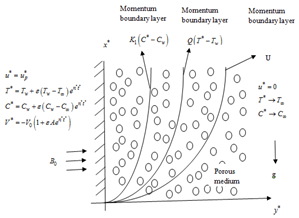Figure 1. Physical model of the problem

$\frac{\partial v^{*}}{\partial y^{*}}=0$     (1)

\frac{\partial u^{*}}{\partial y^{*}}+v^{*} \frac{\partial u^{*}}{\partial y^{*}}=v \frac{\partial^{2} u^{*}}{\partial y^{* 2}}-\left(\frac{\sigma B_{0}^{2}}{\rho}+\frac{v}{K^{*}}\right) u^{*}+g \beta_{T}\left(T^{*}-T_{\infty}\right)+g \beta_{C}\left(C^{*}-C_{\infty}\right)-k_{0}\left(\frac{\partial^{3} u^{*}}{\partial t^{*} \partial y^{* 2}}+v^{*} \frac{\partial^{3} u^{*}}{\partial y^{* 3}}\right) (2) \frac{\partial T^{*}}{\partial t^{*}}+v^{*} \frac{\partial T^{*}}{\partial y^{*}}=\frac{k}{\rho C_{p}} \frac{\partial^{2} T^{*}}{\partial y^{*^{2}}}-\frac{1}{\rho C_{p}} \frac{\partial q_{r}^{*}}{\partial y^{*}}-\frac{Q_{0}}{\rho C_{p}}\left(T^{*}-T_{\infty}\right) (3) \frac{\partial C^{*}}{\partial t^{*}}+v^{*} \frac{\partial C^{*}}{\partial y^{*}}=D \frac{\partial^{2} C^{*}}{\partial y^{*^{2}}}-k_{1}\left(C^{*}-C_{\infty}\right) (4) The boundary conditions for the above described model u^{*}=u_{p^{*}}, T^{*}=T_{w}+\varepsilon\left(T_{w}-T_{\infty}\right) e^{n t^{*}}, C^{*}=C_{w}+\varepsilon\left(C_{w}-C_{\infty}\right) e^{n^{*} t^{*}}, at y^{*}=0 u^{*}=0, T^{*} \rightarrow T_{\infty}, C^{*} \rightarrow C_{\infty} at y^{*} \rightarrow \infty (5) It is unambiguous that Eq. (1) that the velocity of suction at the surface plate is time function. Presuming it yields into the form: v^{*}=-V_{0}\left(1+\varepsilon A e^{n^{*} t^{*}}\right) (6) \varepsilon and A are small such that \varepsilon<<1, A<<1. Acknowledging a self-similar solution of the form u=\frac{u^{*}}{V_{0}}, u=\frac{v^{*}}{V_{0}}, y=\frac{V_{0} y^{*}}{v}, t=\frac{V_{0}^{2} y^{*}}{v}, u_{p}=\frac{u_{p}^{*}}{V_{0}} n=\frac{n^{*} v}{V_{0}^{2}}, \theta=\frac{T^{*}-T_{\infty}}{T_{w}-T_{\infty}}, C=\frac{C^{*}-C_{\infty}}{C_{w}-C_{\infty}} (7) the basic field Eqns. (2) to (4) can be expressed in non- dimensional form as \begin{aligned} \frac{\partial u}{\partial t}-\left(1+\varepsilon A e^{n t}\right) \frac{\partial u}{\partial y} &=\frac{\partial^{2} u}{\partial y^{2}}-\left(M+\frac{1}{K}\right) u+G r \theta+G m C \\ &-E\left[\frac{\partial^{3} u}{\partial t \partial y^{2}}-\left(1+\varepsilon A e^{n t}\right) \frac{\partial^{3} u}{\partial y^{3}}\right] \end{aligned} (8) \frac{\partial \theta}{\partial t}-\left(1+\varepsilon A e^{n t}\right) \frac{\partial \theta}{\partial y}=\frac{1}{\operatorname{Pr}} \frac{\partial^{2} \theta}{\partial y^{2}}-(Q+R) \theta (9) \frac{\partial C}{\partial t}-\left(1+\varepsilon A e^{n t}\right) \frac{\partial C}{\partial y}=\frac{1}{S c} \frac{\partial^{2} C}{\partial y^{2}}-K r C (10) u=u_{p}, \theta=1+\varepsilon e^{n t}, C=1+\varepsilon e^{n t} at y=0 u \rightarrow 0, \theta \rightarrow 0, C \rightarrow 0 as y \rightarrow \infty (11) G r=\frac{\left(T_{w}-T_{\infty}\right) \beta_{T} g v}{V_{0}^{3}}, G m=\frac{\left(C_{w}-C_{\infty}\right) \beta_{C} g v}{V_{0}^{3}} R=\frac{4 v}{\rho C_{p} V_{0}^{2}}, \operatorname{Pr}=\frac{\rho C_{p} v}{k}, K=\frac{K^{*} V_{0}^{2}}{v^{2}}, K r=\frac{K_{1} v}{V_{0}^{2}} S c=\frac{v}{D}, Q=\frac{v Q_{0}}{\rho C_{p} V_{0}^{2}}, E=\frac{k_{0} V_{0}^{2}}{v^{2}} (12) 3. Problem Solution Solutions of Eqns. (8) to (10) are reaped by regular and multi-parameter perturbation technique. E, ε and A are presumed small, such that E <<1 and ε<<1. For getting solutions we introduce \left.\begin{array}{l}u(y, t)=u_{0}(y)+\varepsilon e^{n t} u_{1}(y)+0\left(\varepsilon^{2}\right) \\ \theta(y, t)=\theta_{0}(y)+\varepsilon e^{n t} \theta_{1}(y)+0\left(\varepsilon^{2}\right) \\ C(y, t)=C_{0}(y)+\varepsilon e^{n t} C_{1}(y)+0\left(\varepsilon^{2}\right)\end{array}\right\} (13) where, u_{0}, is the mean velocity, \theta_{0} is the mean temperature and C_{0} is the mean concentration. Applying Eq. (13) into Eqns. (8) to (10). Tallying non-harmonic and harmonic statement to above location, after neglecting coefficient of \varepsilon^{2} we secure zero order E u_{0}^{\prime \prime \prime}+u_{0}^{\prime \prime}+u_{0}^{\prime}-n_{1} u_{0}=-G r \theta_{0}-G m C_{0} (14) \theta_{0}^{\prime \prime}+\operatorname{Pr} \theta_{0}^{\prime}-n_{3} \operatorname{Pr} \theta_{0}=0 (15) C_{o}^{\prime \prime}+S c C_{0}^{\prime}-S c K r C_{0}=0 (16) where, n_{1}=M+\frac{1}{K}, n_{3}=Q+R With u_{0}=u_{p}, \theta_{0}=1, C_{0}=1 at y=0 u_{0} \rightarrow 0, \theta_{0} \rightarrow, C_{0} \rightarrow 0 as y \rightarrow \infty (17) And first order E u_{1}^{\prime \prime \prime}+(1-n E) u_{1}^{\prime \prime}+u_{1}^{\prime}-n_{2} u_{1}=-G r \theta_{1}-G m C_{1}-A E u_{0}^{\prime \prime \prime}-A u_{0}^{\prime}     (18)

$\theta_{1}^{\prime \prime}+\operatorname{Pr} \theta_{1}^{\prime}-n_{4} \operatorname{Pr} \theta_{1}=-\operatorname{Pr} A \theta_{0}^{\prime}$     (19)

$C_{1}^{\prime \prime}+S c C_{1}^{\prime}-S c n_{5} C_{1}=-A S c C_{0}^{\prime}$     (20)

where, $n_{2}=\left(M+\frac{1}{K}+n\right), n_{4}=Q+R+n, n_{5}=K r+n$.

With corresponding boundary conditions

$u_{1}=0, \theta_{1}=1, C_{1}=1$

at $y=0$ $u_{1} \rightarrow 0, \theta_{1} \rightarrow, C_{1} \rightarrow 0$

as $y \rightarrow \infty$     (21)

Eqns. (14) and (18) are differential equations of 3rd order by virtue of visco-elastic parameter. Since there are exclusively two accessible boundary conditions, it necessitates an additional boundary condition to novel solution.

$u_{0}(y)=u_{00}(y)+E u_{01}(y)+0\left(E^{2}\right)$

$u_{1}(y)=u_{10}(y)+E u_{11}(y)+0\left(E^{2}\right)$     (22)

Put Eq. (22) in Eq. (14). Now compare the coefficient of first and zero order of E, Ne can procure

$u_{00}^{\prime \prime}+u_{00}^{\prime}-n_{1} u_{00}=-G r \theta_{0}-G_{m} C_{0}$     (23)

$u_{01}^{\prime \prime}+u_{01}^{\prime}-n_{1} u_{01}=-u_{00}^{\prime \prime \prime}$     (24)

The boundary conditions are

$u_{00}=u_{p}, u_{01}=0,$ on $\quad y=0$

$u_{00} \rightarrow 0, u_{01} \rightarrow 0,$ as $\quad y \rightarrow \infty$     (25)

Put Eq. (22) in Eq. (18) and compare the coefficients of zero and first order of E,

We get

$u_{10}^{\prime \prime}+u_{10}^{\prime}-n_{2} u_{10}=-G r \theta_{01}-G_{m} C_{1}-A u_{00}^{\prime}$     (26)

$u_{11}^{\prime \prime}+u_{11}^{\prime}-n_{2} u_{11}=-A u_{00}^{\prime \prime}-A u_{01}^{\prime}-u_{10}^{\prime \prime \prime}+n u_{10}^{\prime \prime}$     (27)

with

$u_{10}=0, u_{11}=0$

$u_{10} \rightarrow 0, u_{11} \rightarrow 0$

on $y=0$

as $y \rightarrow \infty$     (28)

Using the Eqns. (25) and (28) one can solve the Eqns. (23), (24), (26), and (27) in order to obtain

$u_{00}=A_{7} e^{-m_{5} y}-A_{5} e^{-m_{3} y}-A_{6} e^{-m_{1} y}$

$u_{01}=A_{11} e^{-m_{6} y}+A_{8} e^{-m_{5} y}-A_{9} e^{-m_{3} y}-A_{10} e^{-m_{1} y}$

$u_{10}=A_{17} e^{-m_{7} y}+A_{12} e^{-m_{5} y}-A_{13} e^{-m_{4} y}-A_{14} e^{-m_{3} y}$

$-A_{15} e^{-m_{2} y}-A_{16} e^{-m_{1} y}$

$u_{11}=A_{25} e^{-m_{8} y}+A_{18} e^{-m_{7} y}+A_{19} e^{-m_{6} y}+A_{20} e^{-m_{5} y}-A_{21} e^{-m_{4} y}$

$-A_{22} e^{-m_{3} y}-A_{23} e^{-m_{2} y}-A_{24} e^{-m_{1} y}$

$u_{0}(y)=u_{00}(y)+E u_{01}(y)$

$u_{0}(y)=\left(A_{7} e^{-m_{5} y}-A_{5} e^{-m_{3} y}-A_{6} e^{-m_{1} y}\right)$

$+E\left(A_{11} e^{-m_{6} y}+A_{8} e^{-m_{5} y}-A_{9} e^{-m_{3} y}-A_{10} e^{-m_{1} y}\right)$

$u_{1}(y)=u_{10}(y)+E u_{11}(y)$

$u_{1}(y)=\left(\begin{array}{l}A_{17} e^{-m_{7} y}+A_{12} e^{-m_{6} y}-A_{13} e^{-m_{4} y} \\ -A_{14} e^{-m_{3} y}-A_{15} e^{-m_{2} y}-A_{16} e^{-m_{1} y}\end{array}\right)$

$+E\left(\begin{array}{c}A_{25} e^{-m_{8} y}+A_{18} e^{-m_{7} y}+A_{19} e^{-m_{6} y}+A_{20} e^{-m_{5} y} \\ -A_{21} e^{-m_{4} y}-A_{22} e^{-m_{3} y}-A_{23} e^{-m_{2} y}-A_{24} e^{-m_{1} y}\end{array}\right)$

$u(y, t)=u_{0}(y)+\varepsilon e^{n t} u_{1}(y)$     (29)

$C(y, t)=e^{-m_{1} y}+\varepsilon e^{n t}\left(A_{2} e^{-m_{2} y}+A_{1} e^{-m_{1} y}\right)$     (30)

$\theta(y, t)=e^{-m_{3} y}+\varepsilon e^{n t}\left(A_{4} e^{-m_{4} y}+A_{3} e^{-m_{3} y}\right)$     (31)

Non-dimensional skin friction coefficient Cf, heat transfer rate and mass transfer rates are

$\tau=\left(\frac{\partial u}{\partial y}\right)_{y=0}=\left[\begin{array}{l}\left(-m_{5} A_{7}+m_{3} A_{5}+m_{1} A_{6}\right) \\ +E\left(-m_{6} A_{11}-m_{5} A_{8}+m_{3} A_{9}+m_{1} A_{10}\right)\end{array}\right]$$-\varepsilon e^{n t}\left[\begin{array}{l}-m_{7} A_{17}-m_{5} A_{12}+m_{4} A_{13}+m_{3} A_{14} \\ +m_{2} A_{15}+m_{1} A_{16} \\ +E\left(-m_{8} A_{25}-m_{7} A_{18}-m_{6} A_{19}-m_{5} A_{20}+m_{4} A_{21}\right)\end{array}\right]$     (32)

$N u=-\left(\frac{\partial \theta}{\partial y}\right)$ at $(\mathrm{y}=0)=\left(m_{3}\right)+\varepsilon e^{n t}\left(m_{4} A_{4}+m_{3} A_{3}\right)$     (33)

$S h=-\left(\frac{\partial C}{\partial y}\right)$ at $(\mathrm{y}=0)=\left(m_{1}\right)+\varepsilon e^{n t}\left(m_{2} A_{2}+m_{1} A_{1}\right)$     (34)

4. Results and Discussions

Selected computations have been depicted graphically in all the figures by assigning the values to the pertinent parameters characterizing the fluid flow mechanism. Extensive analytical computations are done for velocities, thermal and concentration distributions together with friction factor feature, Nusselt as well as Sherwood number for distinct standards of physical constraints which illustrate the structures of flow. Numerical conclusions are well established in Figures 2 to 10 additionally Tables 1 to 3.

## 2.png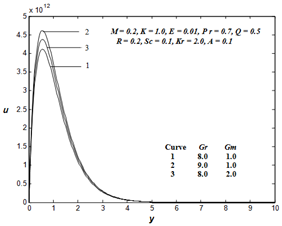Figure 2. Distribution of u for Gr & Gm

Figure 2, illustrates the impact of the flow for heterogeneous values of Grashof number Gr and modified Grashof number Gm. The figure conveys that, Grashof number Gr, modified Grashof number Gm are enlarged, it is perceived that the velocity elevated in general. Also, it is observed that as we shift away from the plate it is perceived that the effect of Grashof number Gr, modified Grashof number Gm are spotted to be not that notable. Figure 3, illustrates the end result of magnitic field parameter M and permeability parameter K on velocity profile. It is fascinating to note from figure that the repercussion of magnetic field is to slow down the value of the velocity profile. The spire value radically declines with raise in the value of the magnetic field, because, the existence of magnetic field incites a force called the Lorentz force. It is also noticed from Figure 3 that the velocity of the flow accelerates with the increase of permeability parameter K.

## 3.png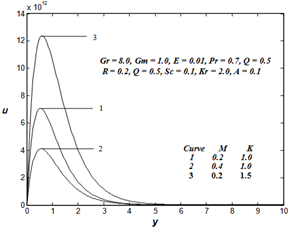Figure 3. Distribution of u for M & K

## 4.pngFigure 4. Distribution of for Pr

Figures 4 and 8 display the dimensionless flow of the fluid and thermal boundary profiles for different values of Pr. These figures explore that the fluid flow and thermal boundary de-escalate with uplift in Pr. This is due to the fact that with the raising values of Pr, thermal conductivity decreases, thus the velocity and temperature decreases with an increase in Pr.

Figures 5 and 9, show the antecedent profiles for assorted values of heat sink parameter Q and radiation R. The fluid flow and temperature profiles decrease with the increase of Q and the fluid flow and temperature profiles are increased with the increase of radiation parameter R. Figure 6 displays the dimensionless velocity for different values of Schmidt number Sc. We observe in this figure that the velocity profiles are reduced with increasing values of the Schmidt number Sc. The influence of visco-eleastic parameter E and suction parameter A on velocity profiles has been illustrated in Figure 7. It can identify that when E and A increase the velocity profile increases. From Figure 10, it can be seen that concentration distribution is detraining function of Sc. Further, it is seen that Sc does not contribute much to the concentration field as we move far away from the boundary surface. Analogous effect is noted with chemical reaction parameter Kr on concentration profile.

## 5.png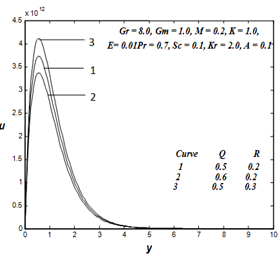Figure 5. Distribution of u for Q & R

## 6.png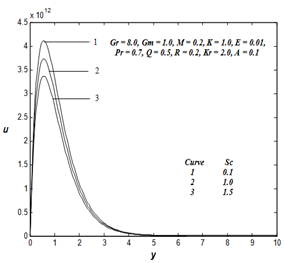Figure 6. Distribution of u for Sc

## 7.png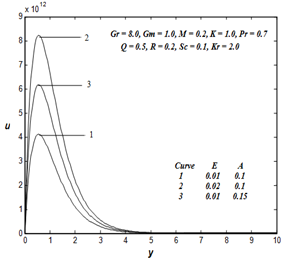Figure 7. Distribution of u for E & A

## 8.png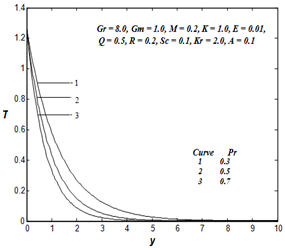Figure 8. Distribution of T for Pr

## 9.pngFigure 9. Distribution of T for Q & R

## 10.png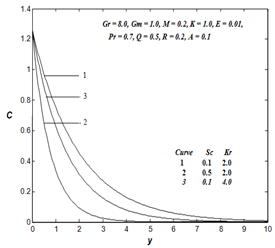Figure 10. Distribution of C for Sc & Kr

The effects of varous parameter such as Grashaf value Gr, modified Grashaf value Gm, magnitic parameter M, porosity parameter K, Prandtl value Pr, radiation parameter R, visco-elastic parameter E, suction parameter A,  heat sink parameter Q, Schmidt value Sc and chemical reaction parameter Kr on the friction factor (Cf), Nusselt value (Nu) and Sherwood value (Sh) are represented in Tables 1 to 3. From the Table 1, It is perceptible that as Grashaf value Gr or modified Grashaf value Gm or porosity parameter K or suction parameter A enhances, the friction factor uplifts, where as the friction factor downtrends as magnetic parameter M or visco-elastic parameter E increases from Table 1. It is concluded that as radiation parameter R or heat sink parameter Q or Prandtl number Pr escalates, it is observed from Table 2 that the friction factor and Nusselt number escalates. From Table 3, it is found that the Sherwood value escalate when both the Schmidth value Sc and chemical reaction parameter Kr accelerate.

Table 1. Impact of different physical parameter on friction factor, Nusselt value and Sherwod value for $P r=0.7, Q=0.0, R=0.2, S c=0.1, K r=2.0$

 Gr Gm M K E A Cf Nu Sh 8.0 1.0 0.2 1.0 0.01 0.1 2.0115 5.7375 0.6432 9.0 2.2585 5.7375 0.6432 10.0 2.5055 5.7375 0.6432 2.0 2.1408 5.7375 0.6432 3.0 2.2702 5.7375 0.6432 0.4 3.6009 5.7375 0.6432 0.6 3.3221 5.7375 0.6432 1.5 5.5941 5.7375 0.6432 2.0 7.8830 5.7375 0.6432 0.02 6.0344 5.7375 0.6432 0.03 4.0230 5.7375 0.6432 0.15 3.0172 5.7375 0.6432 0.17 3.4195 9.7538 0.6432

Table 2. Impact of different physical parameter on friction factor, Nusselt value and Sherwod value for $G r=8.0, G m=K=1.0, M=0.2, E c=0.01, K r=2.0, S c=A=0.1$

 Pr R Q Cf Nu Sh 0.7 0.2 0.5 2.0115 5.7375 0.6432 0.8 2.8576 1.3118 0.6432 1.0 8.7732 3.3588 0.6432 0.8 3.8078 1.8848 0.6432 0.9 4.4935 1.9581 0.6432 1.0 3.3065 1.8110 0.6432 1.5 9.9184 2.1758 0.6432

Table 3. Impact of different physical parameter on friction factor, Nusselt value and Sherwod value for $G r=8.0, G m=1.0, K=1.0, M=0.2, R=0.2, P r=0.7, E c=0.01, K r=2.0$

 Sc Kr Cf Nu Sh 0.1 2.0 2.0115 5.7375 0.6432 0.3 2.0313 5.7375 1.2076 0.5 2.1053 5.7375 1.6467 3.0 2.0124 5.7375 0.7667 4.0 2.0146 5.7375 0.8714
5. Conclusions

In the present study a mathematical model has been developed to simulate 2D unsteady magneto hydrodynamic flow of an incompressible electrically conducting fluid over a permeable moving plate through porous medium under the importance of thermal radiation and chemical reaction. The governed mathematical statement is handled analytically by perturbation technique. The obtained results have led to the following conclusions:

• Fluid velocity is enhancing function of all parameters such as Grashaf number Gr, modified Grashaf number Gm, Permeability parameter K, Radiation parameter R, visco–elastic parameter E, Suction parameter A.
• Thermal boundary distribution falls down against Pr and Q, while radiation parameter R enhances it.
• Presence of chemical reaction enhances the rate of mass transfer which is a desired consequence of the flow of reacting species.
• Friction factor downtrends when magnetic parameter is enlarged. Nussselt number enhances for huge values of Pr. By increasing Schmidt number or chemical reaction parameter Sherwod number progress.
Nomenclature
 $A$ suction parameter $B_{0}$ agnetic field intensity, N. m-1.A-1 $C^{*}$ fluid concentration $C_{\infty}$ free stream dimensional concentration $A$ visco-elastic parameter $D^{*}$ Brownian diffusion coefficient, m2. s-1 $G m$ modified Grashof number $G r$ grashof number $K_{\lambda w}$ absorption coefficient at wall $k^{*}$ permeable parameter $K$ non-dimensional porous parameter $K_{1}$ chemical reaction coefficient $K r$ chemical reaction parameter $M$ magnetic parameter $n^{*}$ constant $N u$ local Nusselt number $P^{*}$ pressure, Pa $P r$ Prandtl number $Q_{0}^{*}$ dimensional heat sink $Q$ heat sink parameter $R$ radiation parameter $R^{*}$ rate of chemical reactive factor $S c$ Schmidt number $S h$ Sherwood number $T^{*}$ Temperature, K $T_{\infty}$ surface temperature, K $T_{\infty}$ free stream dimensional temperature K $U$ stretching velocity, m. s-1 $U_{0}$ reference velocity, m. s-1 $u^{*}, v^{*}$ velocity components in $x^{*}, y^{*}$ directions, m. s-1 $u_{p}^{*}$ wall dimensional velocity, m. s-1 $x^{*}, y^{*}$ Cartesian coordinate in horizontal and vertical directions

Greek symbols

 $v$ kinematic viscosity, m2. s-1 $\varepsilon$ small value $\sigma$ electrical conductivity of the fluid, S. m-1 $\rho$ fluid density, kg. m-3 $v_{0}$ constant suction velocity $\tau_{w}$ surface shear rate, Pa $\beta_{T}$ coefficient of thermal expansion $\beta_{C}$ coefficient of solutal expansion $k$ thermal conductivity of the fluid, W. m-1. k-1 $c_{p}$ specific at constant pressure, J.K-1.kg-1

Appendix

$m_{1}=\frac{S c+\sqrt{S c^{2}+4 S c K r}}{2}, m_{2}=\frac{S c+\sqrt{S c^{2}+4 S c n_{5}}}{2}$,

$m_{3}=\frac{\operatorname{Pr}+\sqrt{\operatorname{Pr}^{2}+4 \operatorname{Pr} n_{3}}}{2}, m_{4}=\frac{\operatorname{Pr}+\sqrt{\operatorname{Pr}^{2}+4 \operatorname{Pr} n_{4}}}{2}$,

$m_{5}=\frac{1+\sqrt{1+4 n_{1}}}{2}, \quad m_{6}=\frac{1+\sqrt{1+4 n_{1}}}{2}, m_{7}=\frac{1+\sqrt{1+4 n_{2}}}{2}$,

$m_{8}=\frac{1+\sqrt{1+4 n_{2}}}{2}, A_{1}=\frac{A S c m_{1}}{m_{1}^{2}-S c m_{1}-S c n_{5}}, A_{2}=1-A_{1}$,

$A_{3}=\frac{A \operatorname{Pr} m_{3}}{m_{3}^{2}-\operatorname{Pr} m 3-\operatorname{Pr} n_{4}}, A_{4}=1-A_{3}, A_{5}=\frac{G r}{m_{3}^{2}-m_{3}-n_{1}}$,

$A_{6}=\frac{G m}{m_{1}^{2}-m_{1}-n_{1}}, A_{7}=u_{p}+A_{5}+A_{6}$,

$A_{8}=\frac{A_{7} m_{5}^{3}}{m_{5}^{2}-m_{5}-n_{1}}, A_{9}=\frac{A_{5} m_{3}^{3}}{m_{3}^{2}-m_{3}-n_{1}}, A_{10}=\frac{A_{6} m_{1}^{3}}{m_{1}^{2}-m_{1}-n_{1}}$,

$A_{11}=A_{10}+A_{9}-A_{8}$,

$A_{12}=\frac{A A_{7} m_{5}}{m_{5}^{2}-m_{5}-n_{2}}, A_{13}=\frac{G r A_{4}}{m_{4}^{2}-m_{4}-n_{2}}$,

$A_{14}=\frac{G r A_{3}+A A_{5} m_{3}}{m_{3}^{2}-m_{3}-n_{2}}, A_{15}=\frac{G r A_{2}}{m_{2}^{2}-m_{2}-n_{2}}$,

$A_{16}=\frac{G m A_{1}+A A_{6} m_{1}}{m_{1}^{2}-m_{1}-n_{2}}, A_{17}=A_{16}+A_{15}+A_{14}+A_{13}-A_{12}$,

$A_{18}=\frac{m_{7}^{3} A_{17}+n A_{17} m_{7}^{2}}{m_{7}^{2}-m_{7}-n_{2}}, A_{19}=\frac{A A_{11} m_{6}}{m_{6}^{2}-m_{6}-n_{2}}$,

$A_{20}=\frac{A A_{7} m_{5}^{3}+A A_{8} m_{5}+A_{12} m_{5}^{3}+n A_{12} m_{5}^{2}}{m_{5}^{2}-m_{5}-n_{2}}$,

$A_{21}=\frac{m_{4}^{3} A_{13}+n A_{13} m_{4}^{2}}{m_{4}^{2}-m_{4}-n_{2}}$,

\begin{aligned} A_{22} &=\frac{A A_{5} m_{3}^{3}+A A_{9} m_{3}+A_{14} m_{3}^{3}+n A_{14} m_{3}^{2}}{m_{3}^{2}-m_{3}-n_{2}} \\ A_{23} &=\frac{m_{2}^{3} A_{15}+n A_{15} m_{2}^{2}}{m_{2}^{2}-m_{2}-n_{2}} \end{aligned},

\begin{aligned} A_{24} &=\frac{A A_{6} m_{1}^{3}+A A_{10} m_{1}+A_{16} m_{1}^{3}+n A_{16} m_{1}^{2}}{m_{1}^{2}-m_{1}-n_{2}} \\ A_{25} &=-A_{19}-A_{18}+A_{24}+A_{23}+A_{22}+A_{21}-A_{20} \end{aligned}.

References

 Beard, D.W., Walters, K. (1964). Elastic-viscous boundary layer flow two dimensional flows near a stagnation point. Mathematical Proceeding of the Camridge Philosophical Society, 60(3): 667-674. https://doi.org/10.1017/S0305004100038147

 Singh, A.K., Gholami, H.R., Soudalgekar, V.M. (1996). Transient free convection flow between two vertical parallel plates. Heat and Mass Transfer, 31(5): 329-331. https://doi.org/10.1007/BF02184046

 Sajid, M., Pop, I., Hayat, T. (2010). Fully developed mixed convection flow of a Visco-elastic fluid between permeable parallel vertical plates. Computers and Mathematics with Applications, 59(1): 493-498. https://doi.org/10.1016/j.camwa.2009.06.017

 Asghar, S., Mohyuddin, M.R., Hayat, T., Siddiqui, A.M. (2004). The flow of a non-Newtonian fluid induced due to the oscillations of a porous plate. Mathematical Problems in Engineering, 2004(2): 133-143. http://dx.doi.org/10.1155/S1024123X04309014

 Hayat. T., Mohyuddin, M.R., Asher, S., Siddiqui, A.M. (2004). Zeitschrift fur angewandte Mathematik und Mechanik, 84(1): 65-70. https://doi.org/10.1002/zamm.200420089

 Chen, C.H. (2004). Heat and mass transfer in MHD flow by natural convection from a permeable, inclined surface with variable wall temperature and concentration. Acta Mechanica, 172(3-4): 219-235. https://doi.org/10.1007/s00707-004-0155-5

 Chamkha, A.J. (2004). Unsteady MHD convective heat and mass transfer past a semi-infinite vertical permeable moving plate with heat absorption. International. Journal of Engineering Science, 45(2): 217-230. https://doi.org/10.1016/S0020-7225(03)00285-4

 Seddeek, M.A., Salama, F.M. (2007). The effect of temperature dependent viscosity and thermal conductivity on unsteady MHD convective heat transfer past a semi-infinite vertical porous plate. Computational Materials Science, 40(2): 186-192. https://doi.org/10.1016/j.commatsci.2006.11.012

 Jha, B.K. (2001). Natural convection in unsteady MHD Couette flow. Heat and Mass Transfer, 37(4): 329-331. https://doi.org/10.1007/PL00013295

 Subhash, A.M., Joshi, A., Sonath, R.M. (2001). Heat transfer in MHD visco-elastic fluid flow over a stretching surface. Zeitschrift für Angewandte Mathematik und Mechanik, 81(10): 691-698. https://doi.org/10.1002/1521-4001(200110)81:10<691::AID-ZAMM691>3.0.CO;2-Z

 Sivaraja, R., Kumar, B.R. (2012). Unsteady MHD dusty Visco-elastic fluid Couette flow in an irregular channel with varying mass diffusion. International Journal of Heat and Mass Transfer, 55(11-12): 3076-3089. https://doi.org/10.1016/j.iheatmass transfer.2012.01.049

 Abel, S., Veena, P.H. (1998). Visco-elastic fluid flow and heat transfer in porous medium over a stretching sheet. International Journal of Non-Linear Mechanics, 33(3): 531-540. https://doi.org/10.1016/S0020-7462(97)00025-5

 Tapas, P.K., Rath, P.K. (2016). Free convective MHD flow of visco-elastic fluid past a vertical porous plate in the presence of heat source and chemical reaction. Journal of Engineering Thermophysics, 25(2): 288-298. https://doi.org/10.1134/S1810232816020132

 Mbeledogu, I.U., Ogulu, A. (2007). Heat and mass transfer of an unsteady MHD natural convection flow of a rotating fluid past a vertical porous plate in the presence of radiative heat transfer. International Journal of Heat and Mass Transfer, 50(9-10): 1902-1908. https://doi.org/10.1016/j.ijheatmasstransfer.2006.10.016

 Suneetha, S., Reddy, N.B., Prasad, V.R. (2011). Radiation and mass transfer effects on MHD free convective dissipative fluid in the presence of heat source/sink. Journal of Applied Fluid Mechanics, 4(1): 107-113.

 Reddy, T.S., Raju, M.C., Varma, S.V.K. (2013). Chemical reaction and radiation effects on MHD free convection flow through a porous medium bounded by a vertical surface with constant heat and mass flux. Journal of Computational and Applied Research in Mechanical Engineering, 3(1): 53-62. https://doi.org/10.22061/jcarme.2013.59

 Ahmed, S. (2010). Influence of chemical reaction on transient MHD free convective flow over a vertical plate in slip-flow Regime. Emirates Journal for Engineering Research, 15(1): 25-34.

 Sandeep, N., Reddy, A.V.B., Sugunamma, V. (2012). Effect of radiation and chemical reaction on transient MHD free convective flow over a vertical plate through porous media. Chemical and Process Engineering Research, 2: 1-10.

 Ibrahim, F.S., Elaiw, A.M., Bak, A.A. (2008). Effect of the chemical reaction and radiation absorption on the unsteady MHD free convection flow past a semi-infinite vertical permeable moving plate with heat source and suction. Communication in Non-linear Science and Numerical Simulation, 13(6): 1056-1066. https://doi.org/10.1016/j.cnsns.2006.09.007

 Ramesh Babu, K., Venkateswarlu, B., Satya Narayana, P.V. (2016). Effects of chemical reaction and radiation on MHD flow of a viscous fluid in a vertical channel with non-uniform concentration. International Journal of Mathematics and Computational Science, 2(2): 34-42.

 Venkateswarlu, B., Satya Narayana, P.V. (2015). MHD viscoelastic fluid flow over a continuously moving vertical surface with chemical reaction. Walailak Journal of Science and Technology, 12(9): 775-783. https://doi.org/10.14456/WJST.2015.54

 Ramesh Babu, K., Venkateswarlu, B., Satya Narayana, P.V. (2014). Effects of chemical reaction and radiation absorption on mixed convective flow in a circular annulus at constant heat and mass flux. Advances in Applied Science Research, 5(5): 122-138.

 Satya Narayana, P.V., Venkateswarlu, B., Venkataramana, S. (2013). Chemical reaction and Radiation absorption effects on MHD micropolar fluid past a vertical porous plate in a rotating system. Journal of Energy, Heat and Mass Transfer, 35(3): 197-214.

 Saxena, S.S., Dubey, G.K. (2011). MHD free convection heat and mass transfer effects flow of visco-elastic fluid embedded in porous medium with variable permeability in the presence of radiation and heat source in slip flow regime. Advances in Applied Science Research, 2(5): 115-129.

 Choudhury, R., Purkayastha, S. (2012). Mixed convective MHD flow of visco-elastic fluid past a vertical infinite plate with mass transfer. International Journal of Scientific and Engineering Research, 3(2): 1-7.

 Choudhury, R., Das, U.J. (2012). Visco-elastic effects on free convective three dimensional flow with heat and mass transfer. ISRN Computational Mathematics, 2012: 402037. http://dx.doi.org/10.5402/2012/402037

 Choudhury, R., Purkayastha, S. (2012). Elastico-viscous effects on an oscillatory heat and mass transfer flow past a vertical plate with thermal diffusion and periodic. International Journal of Advanced Scientific and Technical Research, 2(5): 215-232.

 Choudhury, R., Dey, D. (2012). Mixed convective MHD flow with heat and mass transfer of an elastic-viscous fluid from a vertical surface with ohmic heating in presence of radiation. Journal of Fluids and Thermal Sciences, 1(2): 131-143.

 Choudhury, R., Dey, D. (2012). Free convective elasticviscous fluid flow with heat and mass transfer past an inclined plate in slip flow regime. Latin American Applied Research, 42(4): 327-332.

 Choudhury, R., Dhar, P. (2013). Diffusion thermo effects of visco-elastic fluid past a vertical porous surface in presence of magnetic field and radiation. International Journal of Innovative Research in Science, Engineering and Technology, 2(3): 805-812.

 Ibrahim, S.M., Suneetha, K. (2015). Effects of heat generation and thermal radiation on steady MHD flow near a stagnation point on a linear stretching sheet in porous medium and presence of variable thermal conductivity and mass transfer. Journal of Computational and Applied Research in Mechanical Engineering, 4(2): 133-144.

 Ibrahim, S.M., Suneetha, K. (2016). Effects of thermal diffusion and chemical reaction on MHD transient free convection flow past a porous vertical plate with radiation, temperature gradient dependent heat source in slip flow regime. Journal of Computational and Applied Research in Mechanical Engineering, 5(2): 83-95.

 Ibrahim, S.M., Suneetha, K. (2016). Influence of thermo-diffusion and Heat source on MHD Free convective radiating boundary layer of chemically reacting fluid flow in a porous vertical surface. Journal of advances in Applied Mathematics, 1(1): 17-28. https://doi.org/10.22606/jaam.2016.11003

 Ibrahim, S.M., Suneetha, K. (2015). Influence of chemical reaction and heat source on MHD free convection boundary layer flow of radiation absorbing Krushinski fluid in porous medium. Asian journal of Mathematics and Computer Research, 3(2): 87-103.

 Satya Narayana, P.V., Venkateswarlu, B., Devika, B. (2016). Chemical reaction and heat source effects on MHD oscillatory flow in an irregular channel. Ain Shams Engineering Journal, 7(4): 1079-1088. https://doi.org/10.1016/j.asej.2015.07.012

 Satya Narayana, P.V., Venkateswarlu, B., Devika, B. (2017). Effects of chemical reaction and heat source on MHD oscillatory flow with heat and mass transfer of a viscoelastic fluid in a vertical channel with porous medium. International Journal of Applied Computational Mathematics, 3(1): 937-952. https://doi.org/10.1007/s40819-017-0391-8

 Nayak, M.K., Dash, G.C., Singh, L.P. (2014). Effect of chemical reaction on MHD flow of a visco-elastic fluid through porous medium. Journal of Applied Analysis and Computation, 4(4): 367-381.

 Jena, S., Dash, G.C., Mishra, S.R. (2016). Chemical reaction effect on MHD visco-elastic fluid flow over a stretching sheet with heat source/sink. Ain Shams Engineering Journal, 9(4): 1205-1213. https://doi.org/10.1016/j.asej.2016.06.014

 Babu, K.R., Parandhama, A., Venkateswara Raju, K., Raju, M.C., Sataya Narayana, P.V. (2017). Unsteady MHD free convective flow of a visco-elastic fluid past an infinite vertical porous moving plate with variable temperature and concentration. International Journal of Applied and Computational Mathematics, 3(4): 3411-3431. https://doi.org/10.1007/s40819-017-0306-8